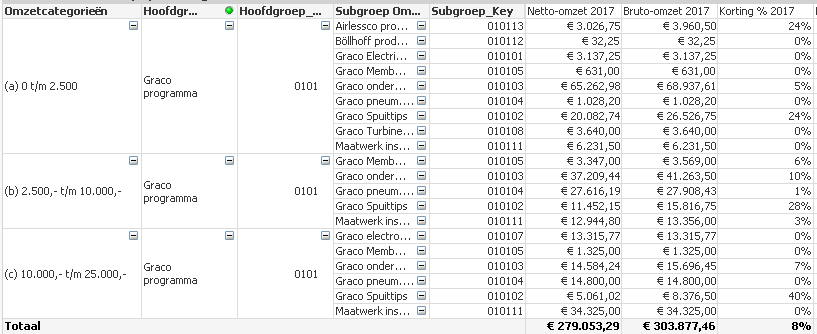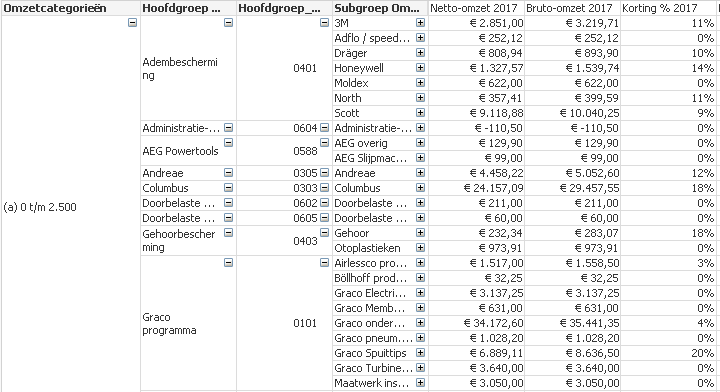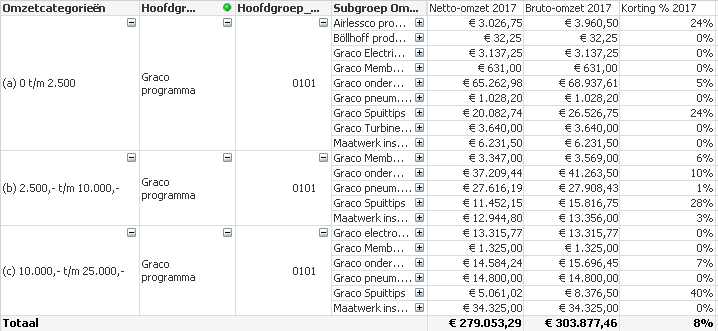# QlikView App Dev

Discussion Board for collaboration related to QlikView App Development.

Announcements
Skip the ticket, Chat with Qlik Support instead for instant assistance.
cancel
Showing results for
Did you mean:Contributor III

## Table expression ignoring my calculated dimension

Hi all,

I need to get a list of discount % on HOOFDGROEP (main-level) and SUBGROEP (sub-level) per category (group of customers).

I defined the categories in a calculated dimension as following:

=if(aggr(sum({\$<Jaar={2017}, Markt={Houtbouw}>}[sales]), Customer)>0 and aggr(sum({\$<Jaar={2017}, Markt={Houtbouw}>}[sales]),Customer)<=2500, '(a) 0 t/m 2.500',

if(aggr(sum({\$<Jaar={2017}, Markt={Houtbouw}>}[sales),Customer)>2500 and aggr(sum({\$<Jaar={2017}, Markt={Houtbouw}>}[sales]),Customer)<=10000, '(b) 2.500,- t/m 10.000,-',

if(aggr(sum({\$<Jaar={2017}, Markt={Houtbouw}>}[sales]),Customer)>10000 and aggr(sum({\$<Jaar={2017}, Markt={Houtbouw}>}[sales),Customer)<=25000, '(c) 10.000,- t/m 25.000,-',

if(aggr(sum({\$<Jaar={2017}, Markt={Houtbouw}>}[sales]),Customer)>25000, '(d) 25.000,- en meer',

if(aggr(sum({\$<Jaar={2017}, Markt={Houtbouw}>}[sales),Customer<=0, '(x) 0 of negatieve omzet')))))

This function is allocating our customers, based on the sum(sales), in each category.

For example, this results in the following table:To this point, my calculated dimension is working fine, showing the sales (netto-omzet 2017), per Hoofdgroep and Subgroep.

However, if I make an additional selection (in this case: Graco onder...) on Subgroep (sub-level) my calculated dimension is not working anymore. As shown in the figure below:The calculated dimension (categories) are shifting, resulting in different sales number which are not reflecting reality.

Netto-omzet 2017 is defined as: =sum([Sales)

Bruto-omzet 2017 is defined as: =sum([Sales Bruto])

Korting % 2017 is defined as: =(1-(Column(1)/Column(2)))

Can anybody help me how to make this work properly.

1 Solution

Accepted SolutionsMVP

May be try this

=Aggr(

If(Sum({\$<Jaar={2017}, Markt={Houtbouw}, Subgroep>}[sales]) <= 0, '(x) 0 of negatieve omzet',

If(Sum({\$<Jaar={2017}, Markt={Houtbouw}, Subgroep>}[sales]) <= 2500, '(a) 0 t/m 2.500',

If(Sum({\$<Jaar={2017}, Markt={Houtbouw}, Subgroep>}[sales]) <= 10000, '(b) 2.500,- t/m 10.000,-',

If(Sum({\$<Jaar={2017}, Markt={Houtbouw}, Subgroep>}[sales) <= 25000, '(c) 10.000,- t/m 25.000,-',

'(d) 25.000,- en meer'))))

, Customer)

6 RepliesMVP

May be try this

=Aggr(

If(Sum({\$<Jaar={2017}, Markt={Houtbouw}, Subgroep>}[sales]) <= 0, '(x) 0 of negatieve omzet',

If(Sum({\$<Jaar={2017}, Markt={Houtbouw}, Subgroep>}[sales]) <= 2500, '(a) 0 t/m 2.500',

If(Sum({\$<Jaar={2017}, Markt={Houtbouw}, Subgroep>}[sales]) <= 10000, '(b) 2.500,- t/m 10.000,-',

If(Sum({\$<Jaar={2017}, Markt={Houtbouw}, Subgroep>}[sales) <= 25000, '(c) 10.000,- t/m 25.000,-',

'(d) 25.000,- en meer'))))

, Customer)Contributor III
Author

However, it does not deliver the desired result yet.

I did insert the new calculated dimension as you proposed.However, if I select a Hoofdgroep (2nd column) in this case Graco Programma, then this table will pop upWith different sales numbers, it seems like it is shifting the customers to another category. Based on their sales realized in the specific selected HOOFDGROEP, which in this case is Graco Programma. Same will happen if I make selections on Sub-level (Subgroep)

Hope you can help me! Thanks in advance!Specialist

Hi All. Sunny is right and you can try to ignore all selections by replacing the set modifier \$ to 1 if there are another filtersContributor III
Author

You're right. Thanks all!MVP

May be you need to ignore selection in Hoofdgroep also

=Aggr(

If(Sum({\$<Jaar={2017}, Markt={Houtbouw}, Subgroep, Hoofdgroep >}[sales]) <= 0, '(x) 0 of negatieve omzet',

If(Sum({\$<Jaar={2017}, Markt={Houtbouw}, Subgroep, Hoofdgroep >}[sales]) <= 2500, '(a) 0 t/m 2.500',

If(Sum({\$<Jaar={2017}, Markt={Houtbouw}, Subgroep, Hoofdgroep >}[sales]) <= 10000, '(b) 2.500,- t/m 10.000,-',

If(Sum({\$<Jaar={2017}, Markt={Houtbouw}, Subgroep, Hoofdgroep >}[sales) <= 25000, '(c) 10.000,- t/m 25.000,-',

'(d) 25.000,- en meer'))))

, Customer)

or everything

=Aggr(

If(Sum({1<Jaar={2017}, Markt={Houtbouw}>}[sales]) <= 0, '(x) 0 of negatieve omzet',

If(Sum({1<Jaar={2017}, Markt={Houtbouw}>}[sales]) <= 2500, '(a) 0 t/m 2.500',

If(Sum({1<Jaar={2017}, Markt={Houtbouw}>}[sales]) <= 10000, '(b) 2.500,- t/m 10.000,-',

If(Sum({1<Jaar={2017}, Markt={Houtbouw}>}[sales) <= 25000, '(c) 10.000,- t/m 25.000,-',

'(d) 25.000,- en meer'))))

, Customer)Creator

Hi, I met the same issue, I'd like to create a dimension for 3 types according to CY-PY OR Diff. But it only shows the customer who have OR within the month selected. How to show all the customers?

= aggr( if(Sum({1<FY={'CY'},FROZENDATE= {"<=\$(=max(FROZENDATE))"},Month>} ORVALUE)-
Sum({1<FY={'PY'},FROZENDATE= {"<=\$(=addyears(max(FROZENDATE),-1))"},Month>} TOVALUE)>0 ,'With OR Growth',
if((Sum({1<FY={'CY'},FROZENDATE= {"<=\$(=max(FROZENDATE))"},Month>} ORVALUE)-